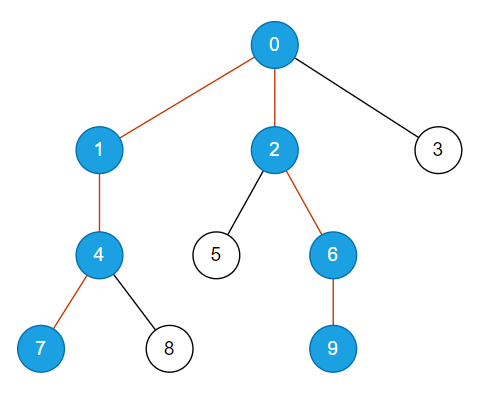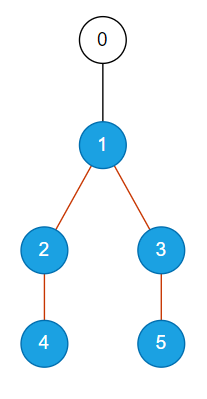2

# Largest Distance Between Two Nodes In A Tree

Difficulty: MEDIUMContributed By
Ankit Kharb
Avg. time to solve
15 min
Success Rate
85%

Problem Statement
Suggest Edit

#### The distance between two nodes is the number of edges in a path between the nodes (there will always be a unique path between any pair of nodes since it is a tree).

##### Note :
``````Use zero-based indexing for the nodes.

The tree is always rooted at 0.
``````
##### Input format :
``````The very first line of input contains an integer ‘T’, denoting the number of test cases.

The first line of each test case contains an integer ‘N’, denoting the number of nodes in the tree.

The next N-1 lines of each test case contain two space-separated integers u and v, denoting an edge between node u and node v.
``````
##### Output format :
``````For each test case, the largest distance between two nodes in the tree is printed.
``````

#### Note :

``````You do not need to print anything, it has already been taken care of. Just implement the given function.
``````
``````Can you solve this problem in just one traversal?
``````
##### Constraints :
``````1 <= T <= 100
2 <= N <= 3000
0 <= u , v < N

Time Limit: 1 sec
``````
##### Sample Input 1 :
``````1
10
0 1
0 2
0 3
1 4
2 5
2 6
4 7
4 8
6 9
``````
##### Sample Output 1 :
``````6
``````
##### Explanation for sample input 1 :
``````For the first test case, the tree is shown below. The longest path in the tree is {7, 4, 1, 0, 2, 6, 9} with a length of 6.
``````##### Sample Input 2 :
``````1
6
0 1
1 2
1 3
2 4
3 5
``````
##### Sample Output 2 :
``````4
``````
##### Explanation for sample input 2 :
``````For the first test case, the tree is shown below. The longest path in the tree is {4, 2, 1, 3, 5} with a length of 4.
``````Console